# CS Decision-Making

C# provides numerous declarations on decision making to help the C # Program flow under certain logical conditions. The following statements are included in the C # decision making.

Decision making structures will require the programmer to specify one or more program conditions for evaluation or testing, together with a statement or statements to be made if the requirement is determined to be true, and optionally, if the situation is determined to be incorrect, other statements will be performed.

• if statement
• if-else statement
• else if statement

This is followed by the typical structure of decision-making in most programming languages: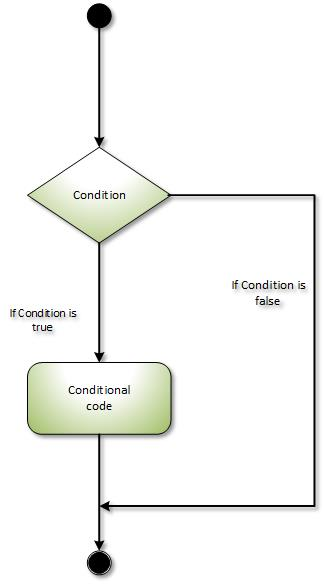The C# if statement checks the condition. If condition is true it is executed.

Syntax:

``````
if(condition){
//code to be executed
}

``````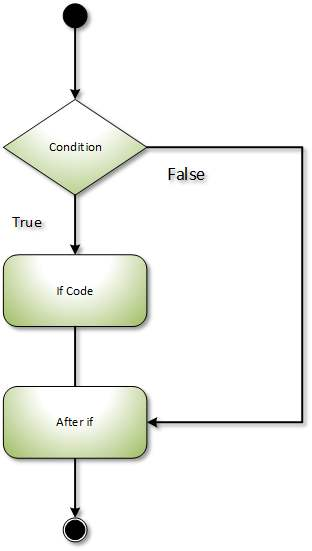Example:

``````
using System;
public class IfExample
{
public static void Main(string[] args)
{
int num = 20;
if (num % 2 == 0)
{
Console.WriteLine("It is even number");
}

}
}

``````

The C# if-else statement also checks the condition. If the condition is true then, if statement execute otherwise (if condition is false), else block statement execute.

Syntax:

``````
if(condition){
//code if condition is true
}else{
//code if condition is false
}

``````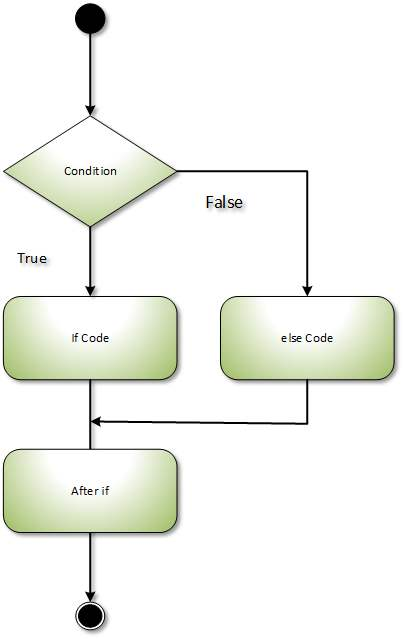Example:

``````
using System;
public class IfExample
{
public static void Main(string[] args)
{
int num = 15;
if (num % 2 == 0)
{
Console.WriteLine("It is even number");
}
else
{
Console.WriteLine("It is odd number");
}

}
}
``````

If we want to check another condition in the else part, the ' If ' statement can follow an ' else ' statement as well.

Syntax:

``````
if(condition1){
//code to be executed if condition1 is true
}else if(condition2){
//code to be executed if condition2 is true
}
else if(condition3){
//code to be executed if condition3 is true
}
...
else{
//code to be executed if all the conditions are false
}

``````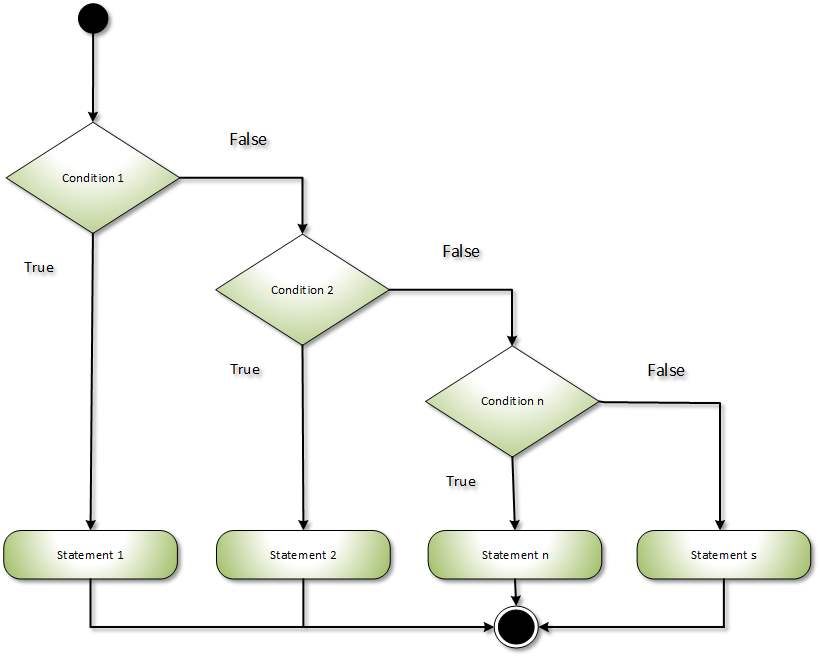Example:

``````
using System;

namespace DecisionMaking {
class Program {
static void Main(string[] args) {
//* local variable definition */
int a = 100;
int b = 200;

/* check the boolean condition */
if (a == 100) {

/* if condition is true then check the following */
if (b == 200) {
/* if condition is true then print the following */
Console.WriteLine("Value of a is 100 and b is 200");
}
}
Console.WriteLine("Exact value of a is : {0}", a);
Console.WriteLine("Exact value of b is : {0}", b);
}
}
}

``````

The switch case in C# execute one statement from several conditions. It is like a if-else-if command in C#.

Syntax:

``````
switch(expression){
case value1:
//code to be executed;
break;
case value2:
//code to be executed;
break;
......

default:
//code to be executed if all cases are not matched;
break;
}

``````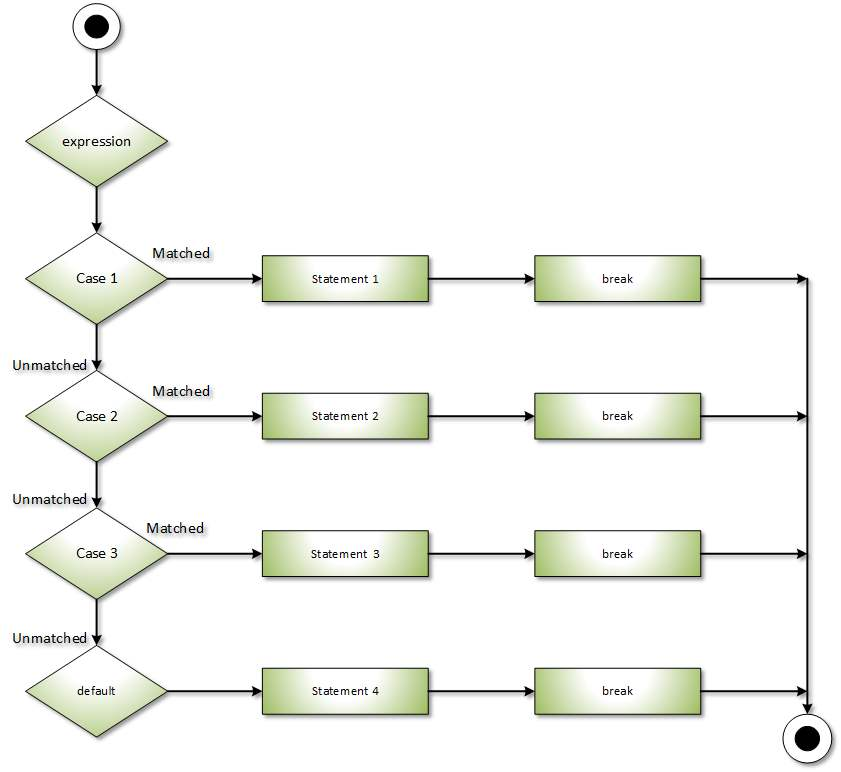Example:

``````
using System;

namespace SwitchCaseExample {
class Program {
static void Main(string[] args) {
/* local variable definition */

case 'A':
Console.WriteLine("Excellent!");
break;
case 'B':
case 'C':
Console.WriteLine("Well Done");
break;
case 'D':
Console.WriteLine("You Passed");
break;
case 'F':
Console.WriteLine("Better Try Again");
break;
default: